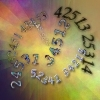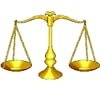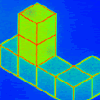# Search by Topic

#### Resources tagged with Place value similar to What Do You Need?:

Filter by: Content type:
Age range:
Challenge level:

### There are 75 results

Broad Topics > Numbers and the Number System > Place value### What Do You Need?

##### Age 7 to 11 Challenge Level:

Four of these clues are needed to find the chosen number on this grid and four are true but do nothing to help in finding the number. Can you sort out the clues and find the number?### Becky's Number Plumber

##### Age 7 to 11 Challenge Level:

Becky created a number plumber which multiplies by 5 and subtracts 4. What do you notice about the numbers that it produces? Can you explain your findings?### Which Is Quicker?

##### Age 7 to 11 Challenge Level:

Which is quicker, counting up to 30 in ones or counting up to 300 in tens? Why?### Digit Sum

##### Age 11 to 14 Challenge Level:

What is the sum of all the digits in all the integers from one to one million?### Subtraction Surprise

##### Age 7 to 14 Challenge Level:

Try out some calculations. Are you surprised by the results?### Arrange the Digits

##### Age 11 to 14 Challenge Level:

Can you arrange the digits 1,2,3,4,5,6,7,8,9 into three 3-digit numbers such that their total is close to 1500?### Being Resilient - Primary Number

##### Age 5 to 11 Challenge Level:

Number problems at primary level that may require resilience.### X Marks the Spot

##### Age 11 to 14 Challenge Level:

When the number x 1 x x x is multiplied by 417 this gives the answer 9 x x x 0 5 7. Find the missing digits, each of which is represented by an "x" .### The Thousands Game

##### Age 7 to 11 Challenge Level:

Each child in Class 3 took four numbers out of the bag. Who had made the highest even number?### Eleven

##### Age 11 to 14 Challenge Level:

Replace each letter with a digit to make this addition correct.### Skeleton

##### Age 11 to 14 Challenge Level:

Amazing as it may seem the three fives remaining in the following `skeleton' are sufficient to reconstruct the entire long division sum.### Double Digit

##### Age 11 to 14 Challenge Level:

Choose two digits and arrange them to make two double-digit numbers. Now add your double-digit numbers. Now add your single digit numbers. Divide your double-digit answer by your single-digit answer. . . .### Song Book

##### Age 7 to 11 Challenge Level:

A school song book contains 700 songs. The numbers of the songs are displayed by combining special small single-digit cards. What is the minimum number of small cards that is needed?### Which Scripts?

##### Age 7 to 11 Challenge Level:

There are six numbers written in five different scripts. Can you sort out which is which?### Being Collaborative - Primary Number

##### Age 5 to 11 Challenge Level:

Number problems at primary level to work on with others.### Being Curious - Primary Number

##### Age 5 to 11 Challenge Level:

Number problems for inquiring primary learners.### Power Mad!

##### Age 11 to 14 Challenge Level:

Powers of numbers behave in surprising ways. Take a look at some of these and try to explain why they are true.### The Number Jumbler

##### Age 7 to 14 Challenge Level:

The Number Jumbler can always work out your chosen symbol. Can you work out how?### Napier's Bones

##### Age 7 to 11 Challenge Level:

The Scot, John Napier, invented these strips about 400 years ago to help calculate multiplication and division. Can you work out how to use Napier's bones to find the answer to these multiplications?### Digit Addition

##### Age 5 to 11 Challenge Level:

Try out this number trick. What happens with different starting numbers? What do you notice?### Method in Multiplying Madness?

##### Age 7 to 14 Challenge Level:

Watch our videos of multiplication methods that you may not have met before. Can you make sense of them?### Three Times Seven

##### Age 11 to 14 Challenge Level:

A three digit number abc is always divisible by 7 when 2a+3b+c is divisible by 7. Why?### Tis Unique

##### Age 11 to 14 Challenge Level:

This addition sum uses all ten digits 0, 1, 2...9 exactly once. Find the sum and show that the one you give is the only possibility.### Repeaters

##### Age 11 to 14 Challenge Level:

Choose any 3 digits and make a 6 digit number by repeating the 3 digits in the same order (e.g. 594594). Explain why whatever digits you choose the number will always be divisible by 7, 11 and 13.### ABC

##### Age 7 to 11 Challenge Level:

In the multiplication calculation, some of the digits have been replaced by letters and others by asterisks. Can you reconstruct the original multiplication?### Basically

##### Age 11 to 14 Challenge Level:

The number 3723(in base 10) is written as 123 in another base. What is that base?### Oddly

##### Age 7 to 11 Challenge Level:

Find the sum of all three-digit numbers each of whose digits is odd.### Multiply Multiples 2

##### Age 7 to 11 Challenge Level:

Can you work out some different ways to balance this equation?### Multiply Multiples 1

##### Age 7 to 11 Challenge Level:

Can you complete this calculation by filling in the missing numbers? In how many different ways can you do it?### Number Detective

##### Age 5 to 11 Challenge Level:

Follow the clues to find the mystery number.### Round the Three Dice

##### Age 7 to 11 Challenge Level:

What happens when you round these three-digit numbers to the nearest 100?### Multiply Multiples 3

##### Age 7 to 11 Challenge Level:

Have a go at balancing this equation. Can you find different ways of doing it?### Six Times Five

##### Age 11 to 14 Challenge Level:

How many six digit numbers are there which DO NOT contain a 5?### Legs Eleven

##### Age 11 to 14 Challenge Level:

Take any four digit number. Move the first digit to the end and move the rest along. Now add your two numbers. Did you get a multiple of 11?### Being Resourceful - Primary Number

##### Age 5 to 11 Challenge Level:

Number problems at primary level that require careful consideration.### Dicey Addition

##### Age 5 to 11 Challenge Level:

Who said that adding couldn't be fun?### Permute It

##### Age 11 to 14 Challenge Level:

Take the numbers 1, 2, 3, 4 and 5 and imagine them written down in every possible order to give 5 digit numbers. Find the sum of the resulting numbers.### Balance Power

##### Age 11 to 18 Challenge Level:

Using balancing scales what is the least number of weights needed to weigh all integer masses from 1 to 1000? Placing some of the weights in the same pan as the object how many are needed?### Quick Times

##### Age 11 to 14 Challenge Level:

32 x 38 = 30 x 40 + 2 x 8; 34 x 36 = 30 x 40 + 4 x 6; 56 x 54 = 50 x 60 + 6 x 4; 73 x 77 = 70 x 80 + 3 x 7 Verify and generalise if possible.### Just Repeat

##### Age 11 to 14 Challenge Level:

Think of any three-digit number. Repeat the digits. The 6-digit number that you end up with is divisible by 91. Is this a coincidence?### Six Is the Sum

##### Age 7 to 11 Challenge Level:

What do the digits in the number fifteen add up to? How many other numbers have digits with the same total but no zeros?### Trebling

##### Age 7 to 11 Challenge Level:

Can you replace the letters with numbers? Is there only one solution in each case?### What an Odd Fact(or)

##### Age 11 to 14 Challenge Level:

Can you show that 1^99 + 2^99 + 3^99 + 4^99 + 5^99 is divisible by 5?### Not a Polite Question

##### Age 11 to 14 Challenge Level:

When asked how old she was, the teacher replied: My age in years is not prime but odd and when reversed and added to my age you have a perfect square...### Spell by Numbers

##### Age 7 to 11 Challenge Level:

Can you substitute numbers for the letters in these sums?### More Mathematical Mysteries

##### Age 11 to 14 Challenge Level:

Write down a three-digit number Change the order of the digits to get a different number Find the difference between the two three digit numbers Follow the rest of the instructions then try. . . .### All the Digits

##### Age 7 to 11 Challenge Level:

This multiplication uses each of the digits 0 - 9 once and once only. Using the information given, can you replace the stars in the calculation with figures?### Even Up

##### Age 11 to 14 Challenge Level:

Consider all of the five digit numbers which we can form using only the digits 2, 4, 6 and 8. If these numbers are arranged in ascending order, what is the 512th number?### Pupils' Recording or Pupils Recording

##### Age 5 to 14

This article, written for teachers, looks at the different kinds of recordings encountered in Primary Mathematics lessons and the importance of not jumping to conclusions!### Nice or Nasty for Two

##### Age 7 to 14 Challenge Level:

Some Games That May Be Nice or Nasty for an adult and child. Use your knowledge of place value to beat your opponent.